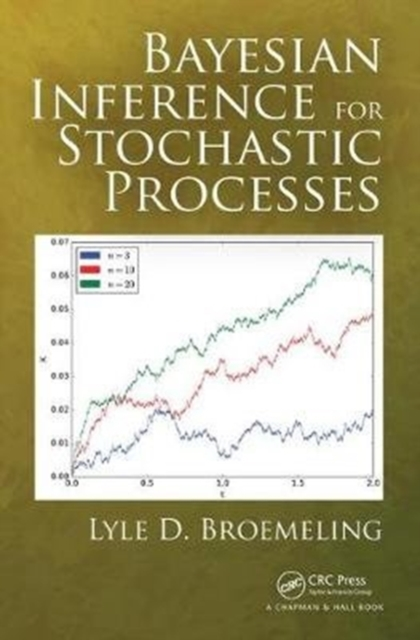# Bayesian Inference for Stochastic Processes Hardback

Hardback

• Information

#### Description

This is the first book designed to introduce Bayesian inference procedures for stochastic processes.

There are clear advantages to the Bayesian approach (including the optimal use of prior information).

Initially, the book begins with a brief review of Bayesian inference and uses many examples relevant to the analysis of stochastic processes, including the four major types, namely those with discrete time and discrete state space and continuous time and continuous state space.

The elements necessary to understanding stochastic processes are then introduced, followed by chapters devoted to the Bayesian analysis of such processes.

It is important that a chapter devoted to the fundamental concepts in stochastic processes is included.

Bayesian inference (estimation, testing hypotheses, and prediction) for discrete time Markov chains, for Markov jump processes, for normal processes (e.g.

Brownian motion and the Ornstein-Uhlenbeck process), for traditional time series, and, lastly, for point and spatial processes are described in detail.

Heavy emphasis is placed on many examples taken from biology and other scientific disciplines.

In order analyses of stochastic processes, it will use R and WinBUGS. Features: Uses the Bayesian approach to make statistical Inferences about stochastic processes The R package is used to simulate realizations from different types of processes Based on realizations from stochastic processes, the WinBUGS package will provide the Bayesian analysis (estimation, testing hypotheses, and prediction) for the unknown parameters of stochastic processes To illustrate the Bayesian inference, many examples taken from biology, economics, and astronomy will reinforce the basic concepts of the subject A practical approach is implemented by considering realistic examples of interest to the scientific community WinBUGS and R code are provided in the text, allowing the reader to easily verify the results of the inferential procedures found in the many examples of the bookReaders with a good background in two areas, probability theory and statistical inference, should be able to master the essential ideas of this book.

£145.00

£116.83